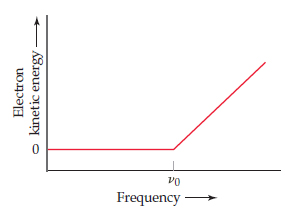# Problem: In an experiment to study the photoelectric effect, a scientist measures the kinetic energy of ejected electrons as a function of the frequency of radiation hitting a metal surface. She obtains the following plot.The point labeled "v0" corresponds to light with a wavelength of 536 nm. Note that when the frequency of the light is greater than v0, the plot shows a straight line with a nonzero slope. What is the slope of this line segment?

###### Problem Details

In an experiment to study the photoelectric effect, a scientist measures the kinetic energy of ejected electrons as a function of the frequency of radiation hitting a metal surface. She obtains the following plot.The point labeled "v0" corresponds to light with a wavelength of 536 nm. Note that when the frequency of the light is greater than v0, the plot shows a straight line with a nonzero slope. What is the slope of this line segment?